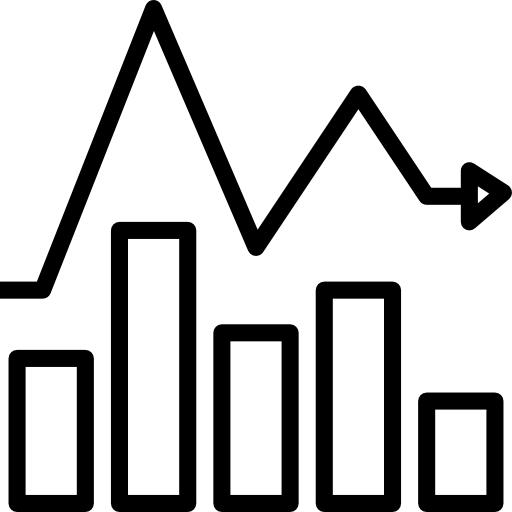1. Introduction The line/bar/pie chart(s)/table(s) + show(s) / exhibit(s) / give(s) information about / compare + how many ___ (the number of) / how much ___ (the amount of) + over a __-year period between ___ and ___ / between ___ and ___ / over ___ years / over the course of ___ years starting in ___. 2. Overview [ Overall / From an overall perspective ] + Major trend:  there (be) an upward/increase trend in ____ / ____ see(s)/saw an upward/increase trend   there (be) a downward/decrease trend in ____/ ____ see(s)/saw a downward/decrease trendthere (be) a fluctuant trend in ____ / ____ illustrate(s) a wave-like movement   Outstanding features: Highest/lowest: _____ (be) the most/least popular /          _____ experience(s/d) the highest/lowest figure.   Others: * The percentage/proportion/number/… of ____ +  in ___ + double(s/d) / (be) 3, 4, 5, … times higher/less than + the figure of that of ____ (e.g. The number of tourists in New York was three times higher than that of Canada.) * A new category of ____ (be) shown / appear(s/ed) in the chart for (year). 3. Body 1 Case 1 (you should group the categories with the same OR contrastive trend in a body and the other is in another body) Case 2 (if no similar/contrastive categories are shown, describe each in turn) Sentence 1 Looking at the details, the number/ratio/percentage… of ____ start(s/ed) / stand(s) / stood at ____ in (year), and then rise(s/rose) / decline(s/d) to reach the highest/lowest point of ___ in (year). Sentence 2 In (year), the figure dip(ped/s) / fall(fell) down significantly / soar(ed/s) / grew considerably to ____. Sentence 3 A similar/contrastive trend (be) recorded in _____ during the period, which goes(went) up / down by ___ from the (year) figure of ___. (e.g. A similar trend was recorded in the number of tourists in Canada during the period, which went up by 5% from the 2005 figure of 15%.) On the other hand/Besides, _____ begin(s/began) at ____ in (year), after that it rise(s/rose) / reduce(s/d) noticeably/slightly in (year) at ____. (e.g. On the other hand, LaGuardia began at 35m in 1995, after that it rose remarkably for the next three years, at 46m.) Sentence 4 ____ was the number/proportion of ____ in (year). In (year), ____ hit(s) the peak/highest point / reach(es/ed) a bottom at ___. 4. Body 2 Sentence 1 The ___ year period witness(es/ed) a ___ climb/fall in the ___, which is(was) ___ in (year 1) and ___ in (year 2). (e.g. The 9 year period witnessed a 9% climb in the percentage of rice produced, which was 35% in 1990 and 44% in 1999.) OR If we look at ____, it start(s/ed) at ____ in the first year. (e.g. However, if we look at Newark airport, it started at the lowest point of 16m passengers in the first year.) Sentence 2 In (year), the figure peak(s/ed) at ____. OR After this point, the trend jump(s/ed) / go(went) down to ____ in (year). Sentence 3 (if any) In the last ___ years, the trend remained stable at ____. OR In the last ___ years, there (be) a wild/mild fluctuation in ___, with the highest and lowest ratio/number shown in (year) at ____ and (year) at ____ respectively.